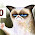## Thursday, September 1, 2016

### ClosedXML: parse excel table based on column names instead of indexes

ClosedXML is a library which provides object oriented way to manipulate Excel 2007/2010 files. I used it in one of the projects to parse table of data from spreadsheet. However, I discovered that there was no way (maybe there is now) to parse the table using the column names. For a while I used column indexes but when the table comes in with rearranged columns the mapping breaks. So I came up with the following solution.

The idea is still to use column indexes and simply generate mapping which helps link column number with column name.

So lets say table looks like this.And the columns are always the same, however they can come in different order. And what's important - some of the columns might have identical names but different data.

And here is table column names as static class. We use it later.

```public static class XlsxColumnNames
{
public const string ColumnA = "Column1";
public const string ColumnB = "Second Column";
public const string ColumnC = "Third Column";
public const string ColumnD = "Column with a long and pointless name";
public const string ColumnDuplicated = "Duplicated Column Name";
}
```

Now we need a model which will represent column name to column index mapping and model to represent Excel table row. But before that lets define some sort of an attribute to mark our model properties: this attribute will contain real name of the column and it will contain a flag which will tell us that this column appears multiple times in Excel table.

```    public class XlsxColumnAttribute : Attribute
{
public string Name { get; set; }

// IsMultiple should be true if there is more than one column with this name
public bool IsMultiple { get; set; }
public XlsxColumnAttribute(string name)
{
Name = name;
}
public XlsxColumnAttribute(string name, bool isMultipe)
{
Name = name;
IsMultiple = isMultipe;
}
}
```

Now back to the actual data and mapping models. I made them both into a single generic class

```public class XlsxRow<T>
{
[XlsxColumn(XlsxColumnNames.ColumnA)]
public T ColumnA { get; set; }

[XlsxColumn(XlsxColumnNames.ColumnB)]
public T ColumnB { get; set; }

[XlsxColumn(XlsxColumnNames.ColumnC)]
public T ColumnC { get; set; }

[XlsxColumn(XlsxColumnNames.ColumnD)]
public T ColumnD { get; set; }

[XlsxColumn(XlsxColumnNames.ColumnDuplicated, IsMultiple = true)]
public List<T> ColumnDuplicated { get; set; }
}
```

Basically, when T is int - this is mapping model which stores index of each column, when T is string - it's actual data model of single row of data from table.
What's left now is to write a couple of helper methods in order to generate mappings for table and to convert data from IXLRow which is ClosedXML type to our XlsxRow model.

Mappings will be generated based on the header table row.

```public static T GetColumnMappings<T>(this IXLRow headerRow) where T : new()
{
var columnMap = new T();

var properties = GetProperties<T>();

foreach (var prop in properties)
{
var attr =
Attribute.GetCustomAttribute(prop, typeof(XlsxColumnAttribute)) as XlsxColumnAttribute;

if (attr == null) continue;

var columnNumbers = cells.Where(
x =>
!x.HasFormula &&
string.Equals(x.GetString().Replace(" ", ""),
attr.Name.Replace(" ", ""),
StringComparison.InvariantCultureIgnoreCase))
.Select(x => x.WorksheetColumn().ColumnNumber())
.ToList();
if (attr.IsMultiple)
{
var list = new List<int>();
foreach (var columnNumber in columnNumbers)
{
}
prop.SetValue(columnMap, list, null);
}
else
{
prop.SetValue(columnMap, columnNumbers.FirstOrDefault(), null);
}

}
return columnMap;
}
```

Here we get column names stored in XlsxColumnAttribute of our model properties, search through header row cells and link column names to column indexes. Getting column mappings will look like this after we found header row:

```var columnMap = header.GetColumnMappings<XlsxRow<int>>();
```

And the last step is to convert ClosedXML row to our data model:

```public static T ConvertRow<T>(this IXLRow row, XlsxRow<int> map) where T : new()
{
var rowMap = new T();

var properties = GetProperties<T>();
foreach (var prop in properties)
{
var attr =
Attribute.GetCustomAttribute(prop, typeof(XlsxColumnAttribute)) as XlsxColumnAttribute;

if (attr == null) continue;

// since mapping and data are instances of the same class XlsxRow<T> property names will be the same
var columnIndex = map.GetType().GetProperty(prop.Name).GetValue(map, null);

if (columnIndex == null)
continue;

if (attr.IsMultiple)
{
var list = new List<string>();
foreach (var index in (List<int>)columnIndex)
{
var cell = row.Cell((int)index);
}
prop.SetValue(rowMap, list, null);
}
else
{
var cell = row.Cell((int)columnIndex);
prop.SetValue(rowMap, cell.GetString(), null);
}

}
return rowMap;
}
```

Here is the usage (dataRow is ClosedXML IXLRow)

```var row = dataRow.ConvertRow<XlsxRow<string>>(columnMap);
```

And here is the source code to play with.

1.1.